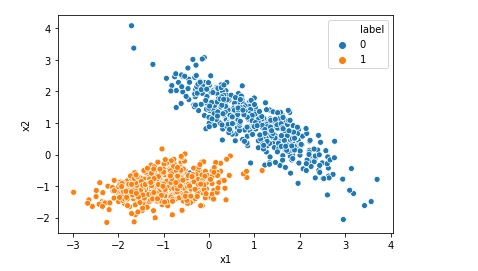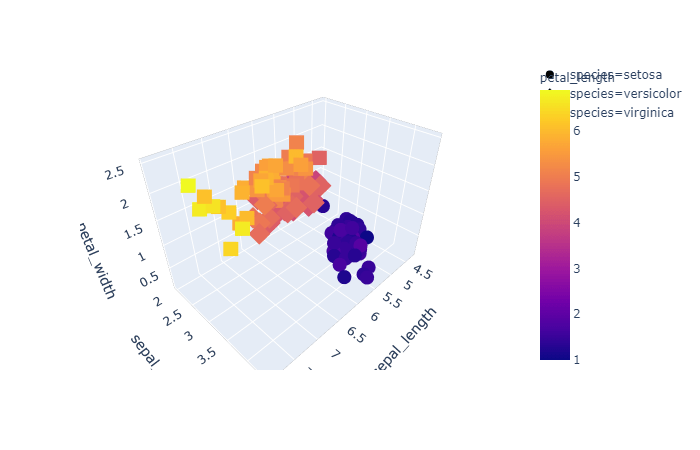# Scatter Plot using Matplotlib in Python

A scatter plot is a type of plot or graph that uses two coordinates axis (say x and y) to display values for two variables for a set of data. We can use matplotlib library of python to create scatter plot in Jupyter notebook. Let us import the required libraries first: #Import the libraries import … Continue reading Scatter Plot using Matplotlib in Python# Difference between Logistic Regression and Support Vector Machine SVM

Both Logistic Regression and Support Vector Machine or SVM can be used for classification however there is some fundamental difference between them. Lets understand those differences when we are using Support Vector Machine as a classifier. Logistic Regression gives probabilities as output that can be interpreted as confidence in the classification decision however Support Vector … Continue reading Difference between Logistic Regression and Support Vector Machine SVM# Top 5 Assumptions of Linear Regression Algorithm

We can not implement Linear Regression on any given data. If we have got a dataset and we are planning to implement Linear Regression Model on that data we must first check if the given data is inline with the assumptions of Linear Regression. Once the given data agrees to all the assumptions then only … Continue reading Top 5 Assumptions of Linear Regression Algorithm

# Top 5 Hyper-Parameters for Logistic Regression

Hyper-parameter is a type of parameter for a machine learning model whose value is set before the model training process starts. Most of the algorithm including Logistic Regression deals with useful hyper parameters. In this post we are going to discuss about the sklearn implementation of hyper-parameters for Logistic Regression. Below is the list of … Continue reading Top 5 Hyper-Parameters for Logistic Regression# Implementing Logistic Regression in 10 lines in Python

Logistic Regression is one of the most popular Machine Learning algorithm used for the classification problems. It should be noted that though there is a regression word in the name of the algorithm Logistic Regression, it is used for classification problems. A use case of Logistic regression could be, based on the symptoms for a disease that a patient has Logistic … Continue reading Implementing Logistic Regression in 10 lines in PythonSupport vector machines or SVM is a supervised machine learning algorithm that can be used for both classification and regression analysis. Advantages of Support Vector algorithm Support vector machine is very effective even with high dimensional data.When you have a data set where number of features is more than the number of rows of data, … Continue reading Top 5 Advantages and Disadvantages of Support Vector Machine AlgorithmDecision Tree is one the most useful machine learning algorithm. Decision tree can be used to solve both classification and regression problem. When we use data points to create a decision tree, every internal node of the tree represents an attribute and every leaf node represents a class label. Like any other machine learning algorithm, … Continue reading Top 6 Advantages and Disadvantages of Decision Tree Algorithm# How to Create 3d Interactive Graph using Plotly

Like matplotlib and seaborn we have plotly, that is a data visualization library used for creating graphical, 3d and interactive graph. You can install plotly using below command pip install plotly Then you can import it in Jupyter notebook as below. import plotly.express as pex Let us use the famous iris dataset to create the … Continue reading How to Create 3d Interactive Graph using Plotly

# Scatter Plot using Lmplot Function of Seaborn

Apart from the methods scatterplot and regplot, seaborn also provides lmplot as another function to draw a scatterplot. However when we create scatter plots using seaborn’s lmplot, it will introduce a regression line in the plot. Let us first import libraries and load the data required to create the plot. import numpy as np import … Continue reading Scatter Plot using Lmplot Function of Seaborn

# Scatter Plot using Regplot Function of Seaborn

Though we have an obvious method named, scatterplot, provided by seaborn to draw a scatterplot, seaborn provides other methods as well to draw scatter plot. One of the other method is regplot. However when we create scatter plots using seaborn's regplot method, it will introduce a regression line in the plot as regplot is based … Continue reading Scatter Plot using Regplot Function of Seaborn